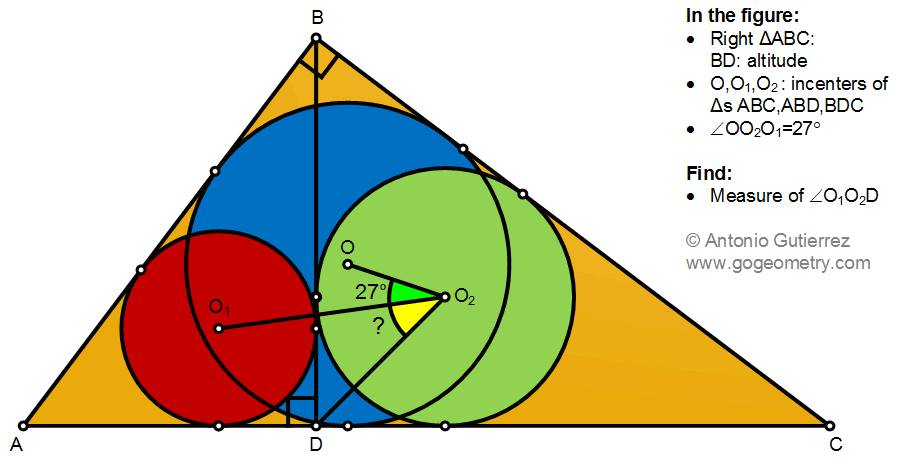## Wednesday, December 30, 2020

### Geometry Problem 1492: Right Triangle, Altitude, Incenters, Angle, Measurement

Geometry Problem 1491. Post your solution in the comment box below.
Level: Mathematics Education, K-12 School, Honors Geometry, College.

Details: Click on the figure below.#### 6 comments:

1.https://photos.app.goo.gl/tKctKLGSK736Hust7
let ∠ (DO2O1)= x
note that C, O and O2 are colinear and ∠ (CDO2)= 45
Triangle ADB and BDC are similar
So DO2/BC=DO1/AB => triangles ABC and O1DO2 are similar ( case SAS)
So ∠ (DO2O1)= ∠ (ACB)
In triangle DO2C, external angle ∠ (OO2D)= x+27=45+x/2
So x= 36 degrees

2.Extend O1O2 to P. From Tr O2PC => Ang C=36, From AngO1O2P = ?+117+27
=> ?=36

1.https://photos.app.goo.gl/iojeJUo4Q3k2kqeC6

3.TR.s AO1D & BO2D are similar having angles 45, A/2, 135 -A/2

So O1D/AD = O2D/BD and so O1D/O2D = AD/BD = (c^2/b)/(ac/b) = c/a
It follows that Tr.s O1DO2 & ABC are similar since < O1DO2 = 90 = <B

Therefore ? = <C and since C/2 + 45 = C + 27,
? = C = 36

Sumith Peiris
Moratuwa
Sri Lanka

4.Join BO and observe that Triangle BOD is similar to CO2D and m(O1DO2)=90
Hence BD/DC=O1D/O2D
but BD/DC=AB/BC => O1DO2 is similar to ABC with m(DO2O1)=C
27+C=45+C/2
=>C=36

1.Correction: Join BO1 and triangle BO1D is similar to CO2D